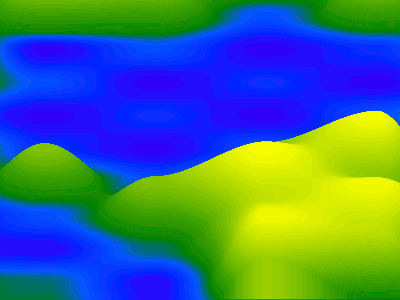• Saves=(a,b,p)=>b*(p*=p*(3-2*p))+a*(1-p);r=X=>S(X^Y);l=_=>s(r(X),r(X+1),X%1);x.drawImage(c,0,-2);for(i=2e3;X=--i/399+9;x.fillRect(i,1078,1,q))q=255*s(l(Y=t),l(Y++),Y%1),x.fillStyle=R(-q,129-q/2,q*3)

267 views

keyboard shortcuts: L or F like